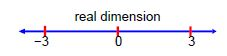Chemistry

# Why use Imaginary numbers and Complex Numbers?

Here giving a longer than normal introduction to imaginary and complex numbers because as a student I couldn’t see why some lecturers and professors wanted to use complex numbers instead of tangents, sines and cosines which I knew from school and also because the explanations in Maths books are not always very helpful. I want to show you why they are so useful, they cut down the use of a calculator and reduce the use of trig relationships. I want to start by comparing imaginary numbers with their close neighbours, the negative numbers. Negative numbers give you a “mirror image” of the positive number on the other side of zero on the number line, thus positive and negative numbers are all one-dimensional. Multiplying x by —1 “flips” you along the number line to —x.For example in words, “I owe the bank £1000 and after doing some evening work I earn £50 and then pay the bank £50, then I now owe the bank £950”. A better, and more elegant way of handling the situation is to use positive and negative numbers and Maths which keep track of the direction along the number line automatically my money = —£1000 +£50 = —£950. You shouldn’t be too smug about the use of negative numbers (don’t say “I know all this, so why bother”, well be patient). Negative numbers were not understood and were considered absurd by many great Mathematicians until the 1700s then they only came into normal usage driven partly by their short elegant way of summarizing things from book keeping to Chemical Analysis.

Imaginary numbers have a similar history to negative numbers, in that people didn’t or still don’t think they are “real” (pun intended). We can solve for x for equations such as x2 = 25 easily, but what does the solution for x2 = —25 mean? Although they may appear strange to us, they are no more strange than zero, negative, fractional and irrational numbers (which can’t be written as a simple fraction) appeared to scientists before us when they were first introduced. Let us assume the imaginary number i exists, where

I = (-1)1/2

As i is just a number (like e and π) it is written in upright roman text. If i exists then Maths becomes easier and new relationships emerge that are easily described. I dislike the term “imaginary number” it was introduced when i was discovered around 1545 by the first group of scientists who thought they were nonsense and absurd. The number i is just as normal as other numbers but the name “imaginary” stuck for purely historical reasons and the terms “real” and “imaginary” are just labels.